# 3 Phase Full Wave Diode Rectifier (Equations And Circuit Diagram)

## What is a Three Phase Full Wave Diode Rectifier?

A three-phase full-wave diode rectifier is obtained by using two half-wave rectifier circuits. The advantage of this circuit is that it produces a lower ripple output than a half-wave 3-phase rectifier. This is because it has a frequency of six times the input AC waveform.

A three-phase full-wave diode rectifier with purely resistive load is shown below. The AC voltage supply is 110 V line to line and 50 Hz frequency.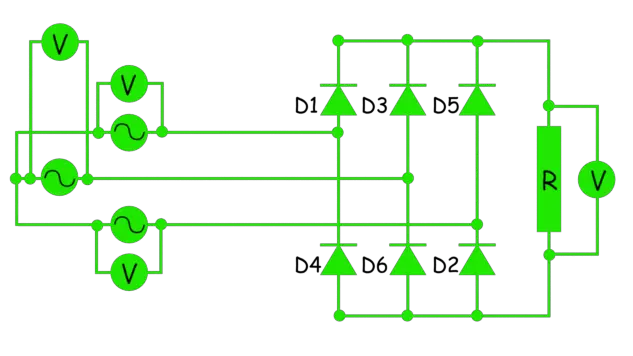The waveform of the voltage across the load is shown in black in the figure below. Vm-phase is the maximum phase voltage. The minimum voltage across the load is 1.5 Vm-phase.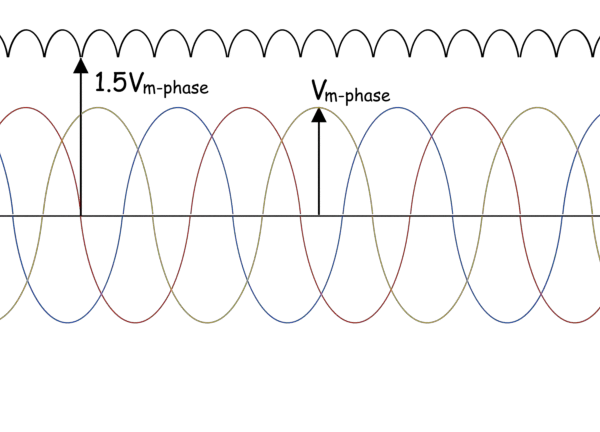From the circuit above, we can see that diode D1 starts to conduct at 30o when the voltage in the R phase is maximum. Diode D1 stops conduction, and diode D3 takes over conduction at angle 150o when the voltage in the Y phase is maximum.

While diode D1 conducts from 30o to 150o in the positive cycle, it is helped by the diode D6, which helps complete the path of return to the 3 phase source. Diode D6 sees the maximum negative voltage, but this remains true only up to 90o when diode D2 takes over conduction from D6 and completes the path along with diode D1.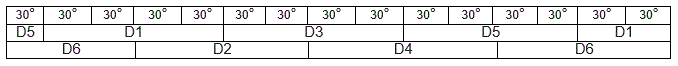• Therefore each diode pair conducts for 60o, and each diode conducts for 120o.
• The load voltage has a ripple, and the frequency of this ripple is 6 × 50 = 300 Hz.

The magnitude of the DC voltage is the sum of the magnitude of the voltages in the two diodes that are conducting at any instant, which is incidentally also a particular line voltage depending on which diode pair is conducting.
The average of the output voltage across the resistive load is given by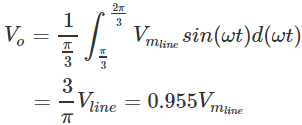where,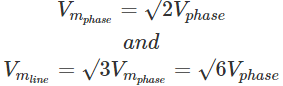The RMS value of the output voltage is given by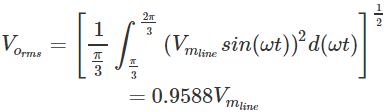Ripple voltage,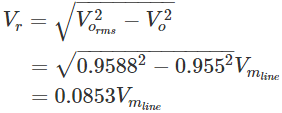Voltage ripple factor,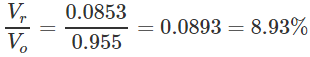DC output power,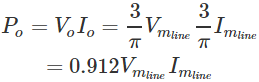AC input power,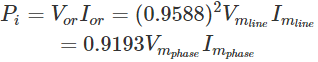Efficiency,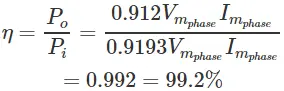The voltage and current across the diode D1 are as shown below.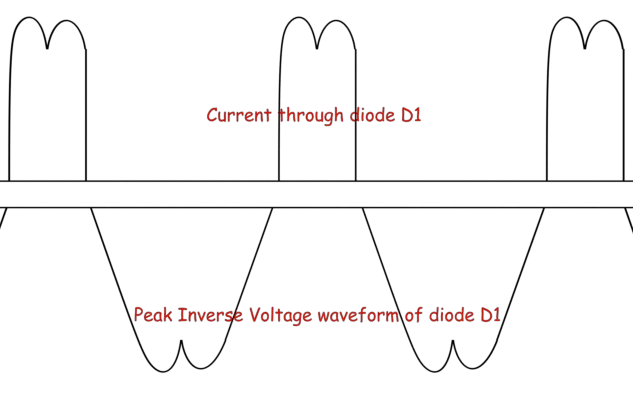We can see from the voltage waveform above that the PIV, Peak Inverse Voltage, which is the maximum voltage faced by the diode when reverse biased, is √3 Vm-phase.

Want To Learn Faster? 🎓
Get electrical articles delivered to your inbox every week.
No credit card required—it’s 100% free.